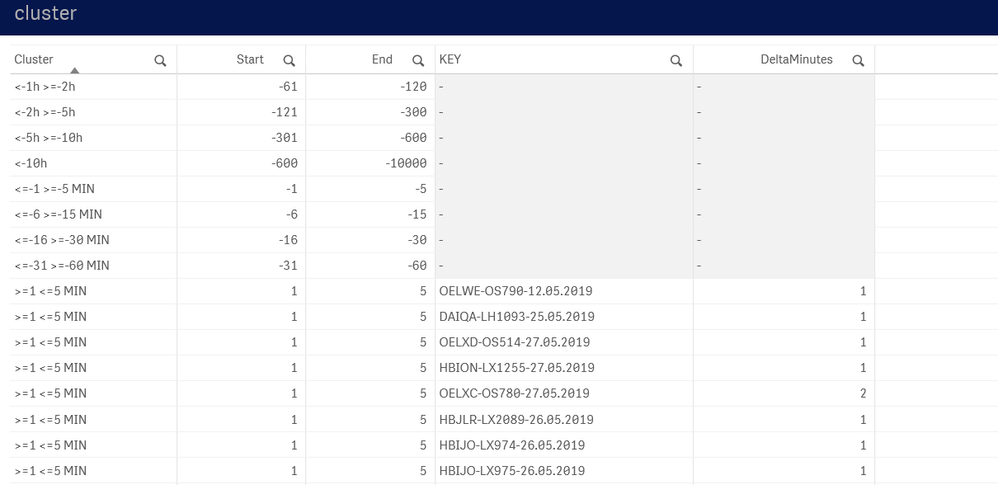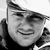Announcements
You can succeed best and quickest by helping others to succeed. Join the conversation.
cancel
Showing results for
Did you mean:Contributor III

## Intervalmatch negative values

Dear folks,

I am trying to use intervalmatch with negative values, as well.

Unfortunately only my positive intervals get matched.

Any ideas?Here is some code sample:

Time_Intervals:

Start, End, Cluster, FR24.event

0, 0, ZERO, gate_arrival

1, 5, >=1 <=5 MIN, gate_arrival
6, 15, >=6 <=15 MIN, gate_arrival
16, 30, >=16 <=30 MIN, gate_arrival
31, 60, >=31 <=60 MIN, gate_arrival
61, 120, >1h <=2h, gate_arrival
121,300, >2h <=5h, gate_arrival
301, 600, >5h <=10h, gate_arrival
600, 10000, >10h, gate_arrival

-1, -5, <=-1 >=-5 MIN, gate_arrival
-6, -15, <=-6 >=-15 MIN, gate_arrival
-16, -30, <=-16 >=-30 MIN, gate_arrival
-31, -60, <=-31 >=-60 MIN, gate_arrival
-61, -120, <-1h >=-2h, gate_arrival
-121,-300, <-2h >=-5h, gate_arrival
-301, -600, <-5h >=-10h, gate_arrival
-600, -10000, <-10h, gate_arrival

0, 0, ZERO, gate_departure

1, 5, >=1 <=5 MIN, gate_departure
6, 15, >=6 <=15 MIN, gate_departure
16, 30, >=16 <=30 MIN, gate_departure
31, 60, >=31 <=60 MIN, gate_departure
61, 120, >1h <=2h, gate_departure
121,300, >2h <=5h, gate_departure
301, 600, >5h <=10h, gate_departure
600, 10000, >10h, gate_departure

-1, -5, <=-1 >=-5 MIN, gate_departure
-6, -15, <=-6 >=-15 MIN, gate_departure
-16, -30, <=-16 >=-30 MIN, gate_departure
-31, -60, <=-31 >=-60 MIN, gate_departure
-61, -120, <-1h >=-2h, gate_departure
-121,-300, <-2h >=-5h, gate_departure
-301, -600, <-5h >=-10h, gate_departure
-600, -10000, <-10h, gate_departure
];

Join(Time_Intervals)
IntervalMatch(DeltaMinutes, FR24.event)
Start,
End,
[FR24.event]
Resident Time_Intervals;

2 Solutions

Accepted SolutionsMVP

You need to switch the values start and end for the negative values in your inline table.

-5 is smaller than -1.Contributor III
Author

great!  That worked!

Is the sorting of the intervals also important?

3 RepliesMVP

You need to switch the values start and end for the negative values in your inline table.

-5 is smaller than -1.Contributor III
Author

great!  That worked!